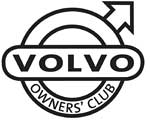# Volvo S90 V90 VIN Plates

1800  |  200  |  300  |  400  |  700  |  850 - 900  |  40/50  |  60  |  70  |  80  |  90  |  XC90

This information will help you to understand the information on the VIN plate of your Volvo car.

Click on the model series number above

Example VIN Number:

YV1X44872T1123456

YV1 X44 87 2 T 1 123456
Manufacturer Code Vehicle Type Engine Type Gearbox Model Year Factory Code Chassis Number

Manufacturer Code
YV1 = cars YV2 = trucks YV3 = buses YV4 = multipurpose 4V1 = trucks 4V2 = 4V3 =
4V4 = trucks 4V5 = trucks 4V6 = 4VL = 4VM = 4VZ = MHA = PT. Central Sole Agency

Engine Type
20 = B200G 21 = B200FT 22 = B200GT 25 = 204FT 27 = B200F 74 = D24TIC 80 = B230G 81 = B230FK 82 = B230GT W/O EGR, W/O TWC 83 = B230FD Pulsair + EGR 84 = B230GK
85 = B230FB 86 = B230FT EGR, TWC 87 = B230FT W/O EGR, TWC 88 = B230F 92 = B6244S 93 = B6254S EGR, TWC 95 = B6304S TWC 96 = B6304S TWC 98 = B6304GS W/O TWC 99 = B6254S W/O EGR, W/O TWC ---

Gearbox
1 = M90 2 = M46 3 = M47 5 = ZF22 6 = "AW" LOCK-UP 7 = "AW" W/O LOCK-UP

Model Year
R = 1994 S = 1995 T = 1996 V = 1997 W = 1998

Factory Code
0 = Sweden, Kalmar Plant 1 = Sweden, Torslanda Plant VCT 21(Volvo Torslandaverken) (Gothenburg) 2 = Belgium, Ghent Plant VCG 22 3 = Canada, Hatdfax Plant 4 = Italy, - Bertone models 240 5 = Malaysia, 6 = Australia,
7 = Indonesia, A = Sweden, Uddevalla Plant (Volvo Cars/TWR (Tom Walkinshaw Racing)) B = Italy, - Bertone Chongq 31 D = Italy, - Bertone models 780 E = Singapore, F = The Netherlands, Born Plant (NEDCAR) J = Sweden, Uddevalla Plant VCU 38 (Volvo Cars/ Pininfarina Sverige AB)

Model Year From 1980
A = 1980 B = 1981 C = 1982 D = 1983 E = 1984 F = 1985 G = 1986 H = 1987 J = 1988 K = 1989 L = 1990 M = 1991
N = 1992 P = 1993 R = 1994 S = 1995 T = 1996 V = 1997 W = 1998 X = 1999 Y = 2000 1 = 2001 2 = 2002 3 = 2003
4 = 2004 5 = 2005 6 = 2006 7 = 2007 8 = 2008 9 = 2009 A = 2010 (code restarted) B = 2011 C = 2012 D = 2013 E = 2014 ---